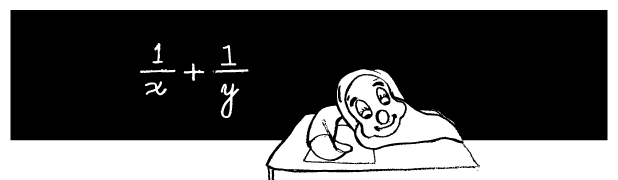Avoid This Common Mistake Made With Fractions

Algebra Level 3Sleepy was sitting in class hearing his teacher talk about fractions. He learns that

$` \frac{x}{n} + \frac{y}{n} = \frac{ (x+y)} { n} \text{ because } x + y = (x+y) '$

before falling asleep. As punishment for this misdemeanor, his teacher made him solve $\frac{1}{x} + \frac{1}{y}$ on the board. Drawing from the previous example, he said that $\frac{ 1}{x} + \frac{1}{y} = \frac{1}{(x+y)}.$

How many ordered pairs of integers subject to $-100 \leq x \leq 100, -100 \leq y \leq 100$ are there, such that

$\frac{ 1}{x} + \frac{1}{y} = \frac{1}{(x+y)}?$

×